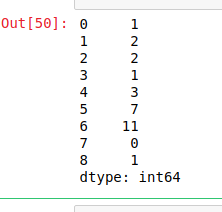Related Articles
Python | Pandas Series.combine()
• Last Updated : 30 Sep, 2019

Python is a great language for doing data analysis, primarily because of the fantastic ecosystem of data-centric Python packages. Pandas is one of those packages and makes importing and analyzing data much easier.

Pandas `Series.combine()` is a series mathematical operation method. This is used to combine two series into one. The shape of output series is same as the caller series. The elements are decided by a function passed as parameter to `combine()` method. The shape of both series has to be same otherwise it will throw an error.

Syntax: Series.combine(other, func, fill_value=nan)

Parameters:
other: other series or list type to be combined with caller series
func: Function passed as parameter which will decide from which series the element should be put at that index
fill_value: integer value of level in case of multi index

Return: Combined series with same shape as caller series

Example #1:

In this example, two lists are made and converted into pandas series using .Series() method. A function is made using lambda which checks which values is smaller in both series and returns whichever is the smaller.

 `# importing pandas module``import` `pandas as pd`` ` `# creating first series``first ``=``[``1``, ``2``, ``5``, ``6``, ``3``, ``7``, ``11``, ``0``, ``4``]`` ` `# creating second series``second ``=``[``5``, ``3``, ``2``, ``1``, ``3``, ``9``, ``21``, ``3``, ``1``]`` ` `# making series``first ``=` `pd.Series(first)`` ` `# making seriesa``second ``=` `pd.Series(second)`` ` `# calling .combine() method``result ``=` `first.combine(second, (``lambda` `x1, x2: x1 ``if` `x1 < x2 ``else` `x2))`` ` `# display``result`

Output:
As shown in the output image, the returned series is having smaller values from both series.Example #2:

In this example, Null values are passed too using `Numpy.nan` method. Since series contains null values, 5 is passed to fill_value parameter to replace null values by 5. A lambda function is passed which will compare values in both series and will return the greater one.

 `# importing pandas module``import` `pandas as pd`` ` `# importing numpy module``import` `numpy as np`` ` `# creating first series``first ``=``[``1``, ``2``, np.nan, ``5``, ``6``, ``3``, np.nan, ``7``, ``11``, ``0``, ``4``, ``8``]`` ` `# creating second series``second ``=``[``5``, ``3``, ``2``, np.nan, ``1``, ``3``, ``9``, ``21``, ``3``, np.nan, ``1``, np.nan]`` ` `# making series``first ``=` `pd.Series(first)`` ` `# making seriesa``second ``=` `pd.Series(second)`` ` `# calling .combine() method``result ``=` `first.combine(second, func ``=``(``lambda` `x1, x2: x1 ``if` `x1 > x2 ``else` `x2), fill_value ``=` `5``)`` ` `# display``result`

Output:
As shown in the output, the NaN values in the series were replaced by 5 before combining the series.

```
0      5.0
1      3.0
2      2.0
3      5.0
4      6.0
5      3.0
6      9.0
7     21.0
8     11.0
9      5.0
10     4.0
11     8.0
dtype: float64```

Attention geek! Strengthen your foundations with the Python Programming Foundation Course and learn the basics.

To begin with, your interview preparations Enhance your Data Structures concepts with the Python DS Course.

My Personal Notes arrow_drop_up